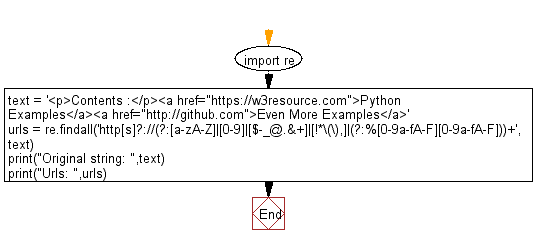﻿ Python: Find urls in a string - w3resource# Python: Find urls in a string

## Python Regular Expression: Exercise-42 with Solution

Write a Python program to find urls in a string.

Sample Solution:-

Python Code:

``````import re
text = '<p>Contents :</p><a href="https://w3resource.com">Python Examples</a><a href="http://github.com">Even More Examples</a>'
urls = re.findall('http[s]?://(?:[a-zA-Z]|[0-9]|[[email protected]&+]|[!*\(\),]|(?:%[0-9a-fA-F][0-9a-fA-F]))+', text)
print("Original string: ",text)
print("Urls: ",urls)
```
```

Sample Output:

```Original string:  Contents :Python ExamplesEven More Examples
Urls:  ['https://w3resource.com', 'http://github.com']
```

Pictorial Presentation:Flowchart:## Visualize Python code execution:

The following tool visualize what the computer is doing step-by-step as it executes the said program:

Python Code Editor:

Have another way to solve this solution? Contribute your code (and comments) through Disqus.

What is the difficulty level of this exercise?

Test your Programming skills with w3resource's quiz.

﻿

## Python: Tips of the Day

Combining two iterable of tuples or pivot nested iterables:

```# Combining two iterables
>>> a = [1, 2, 3]
>>> b = ['a', 'b', 'c']
>>> z = zip(a, b)
>>> z
[(1, 'a'), (2, 'b'), (3, 'c')]

# Pivoting list of tuples
>>> zip(*z)
[(1, 2, 3), ('a', 'b', 'c')]
```